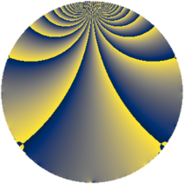# Properties

 Label 129.1.lLevel $129$ Weight $1$ Character orbit 129.l Rep. character $\chi_{129}(11,\cdot)$ Character field $\Q(\zeta_{14})$ Dimension $6$ Newform subspaces $1$ Sturm bound $14$ Trace bound $0$

# Related objects

## Defining parameters

 Level: $$N$$ $$=$$ $$129 = 3 \cdot 43$$ Weight: $$k$$ $$=$$ $$1$$ Character orbit: $$[\chi]$$ $$=$$ 129.l (of order $$14$$ and degree $$6$$) Character conductor: $$\operatorname{cond}(\chi)$$ $$=$$ $$129$$ Character field: $$\Q(\zeta_{14})$$ Newform subspaces: $$1$$ Sturm bound: $$14$$ Trace bound: $$0$$

## Dimensions

The following table gives the dimensions of various subspaces of $$M_{1}(129, [\chi])$$.

Total New Old
Modular forms 18 18 0
Cusp forms 6 6 0
Eisenstein series 12 12 0

The following table gives the dimensions of subspaces with specified projective image type.

$$D_n$$ $$A_4$$ $$S_4$$ $$A_5$$
Dimension 6 0 0 0

## Trace form

 $$6 q - q^{3} - q^{4} - 2 q^{7} - q^{9} + O(q^{10})$$ $$6 q - q^{3} - q^{4} - 2 q^{7} - q^{9} - q^{12} - 2 q^{13} - q^{16} - 2 q^{19} - 2 q^{21} - q^{25} - q^{27} - 2 q^{28} + 5 q^{31} + 6 q^{36} - 2 q^{37} + 5 q^{39} - q^{43} - q^{48} + 4 q^{49} + 5 q^{52} + 5 q^{57} - 2 q^{61} - 2 q^{63} - q^{64} - 2 q^{67} - 2 q^{73} - q^{75} - 2 q^{76} - 2 q^{79} - q^{81} - 2 q^{84} - 4 q^{91} - 2 q^{93} - 2 q^{97} + O(q^{100})$$

## Decomposition of $$S_{1}^{\mathrm{new}}(129, [\chi])$$ into newform subspaces

Label Dim. $$A$$ Field Image CM RM Traces $q$-expansion
$$a_2$$ $$a_3$$ $$a_5$$ $$a_7$$
129.1.l.a $$6$$ $$0.064$$ $$\Q(\zeta_{14})$$ $$D_{7}$$ $$\Q(\sqrt{-3})$$ None $$0$$ $$-1$$ $$0$$ $$-2$$ $$q-\zeta_{14}^{5}q^{3}+\zeta_{14}^{4}q^{4}+(-\zeta_{14}+\zeta_{14}^{6}+\cdots)q^{7}+\cdots$$